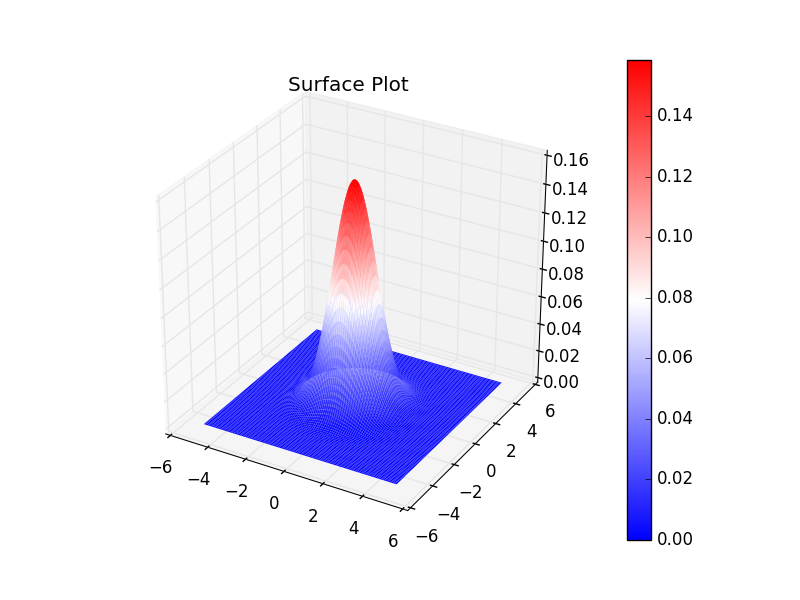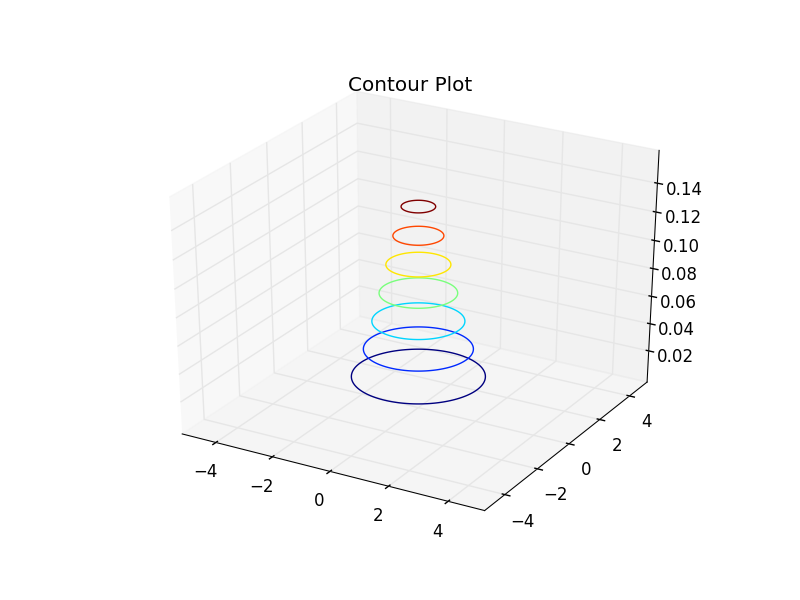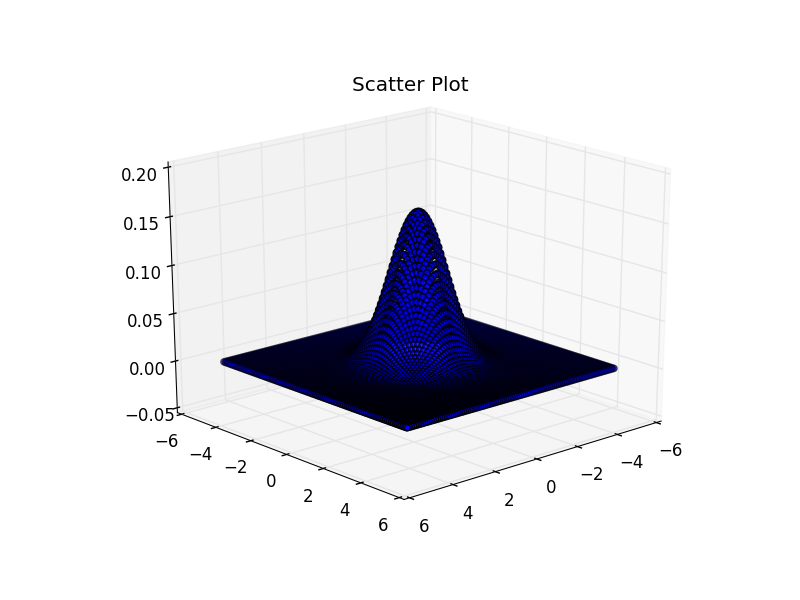# [PYTHON] 3D plot with matplotlib

A brief summary for plotting in 3D with matplotlib. Let's plot the probability density function of the bivariate normal distribution in 3D.

See the official tutorial for details.

# Setting

Import what you need for the time being. Also set the number of dimensions and parameters of the normal distribution.

``````import matplotlib
print(matplotlib.__version__)
# 1.5.1

import numpy as np
from scipy.stats import multivariate_normal

#for plotting
import matplotlib.pyplot as plt
from mpl_toolkits.mplot3d import Axes3D

m = 2 #dimension
mean = np.zeros(m)
sigma = np.eye(m)

``````

There seems to be no major change in the latest version (ver 2.2.2 stable version). See here for details. The mplot3d Toolkit

# Various plots

Surface Plot Try Surface Plot (Surface plot in Japanese?). Note that the data passed to the plot_surface function is a two-dimensional array.

``````N = 1000
x1 = np.linspace(-5, 5, N)
x2 = np.linspace(-5, 5, N)

X1, X2 = np.meshgrid(x1, x2)
X = np.c_[np.ravel(X1), np.ravel(X2)]

Y_plot = multivariate_normal.pdf(x=X, mean=mean, cov=sigma)
Y_plot = Y_plot.reshape(X1.shape)

fig = plt.figure()
ax = fig.add_subplot(111, projection='3d')
surf = ax.plot_surface(X1, X2, Y_plot, cmap='bwr', linewidth=0)
fig.colorbar(surf)
ax.set_title("Surface Plot")
fig.show()

# X1.shape : (1000, 1000)
# X2.shape : (1000, 1000)
# Y_plot.shape : (1000, 1000)

``````Contour Plot Contour Plot can be done in the same way as surface plot.

``````N = 1000
x1 = np.linspace(-5, 5, N)
x2 = np.linspace(-5, 5, N)

X1, X2 = np.meshgrid(x1, x2)
X = np.c_[np.ravel(X1), np.ravel(X2)]
Y_plot = multivariate_normal.pdf(x=X, mean=mean, cov=sigma)
Y_plot = Y_plot.reshape(X1.shape)

fig = plt.figure()
ax = fig.add_subplot(111, projection='3d')
ax.contour(X1, X2, Y_plot)
ax.set_title("Contour Plot")
fig.show()

# X1.shape : (1000, 1000)
# X2.shape : (1000, 1000)
# Y_plot.shape : (1000, 1000)

``````Scatter Plot Unlike before, the data passed to the scatter plot is a one-dimensional array.

``````N = 100
x1 = np.linspace(-5, 5, N)
x2 = np.linspace(-5, 5, N)

X1, X2 = np.meshgrid(x1, x2)
X_plot = np.c_[np.ravel(X1), np.ravel(X2)]

y = multivariate_normal.pdf(X_plot, mean=mean, cov=sigma)

fig = plt.figure()
ax = fig.add_subplot(111, projection='3d')
ax.scatter3D(np.ravel(X1), np.ravel(X2), y)
ax.set_title("Scatter Plot")
plt.show()

# np.ravel(X1).shape : (10000,)
# np.ravel(X2).shape : (10000,)
# y.shape : (10000,)

``````Scatter plot is not for when you want to see the shape of such a function, so it can't be helped that it is hard to see.

Looking at other articles, there are examples of creating ax objects for 3D as follows, but

``````import matplotlib.pyplot as plt
from mpl_toolkits.mplot3d import Axes3D

fig = plt.figure()
ax = Axes3D(fig)
#<class 'mpl_toolkits.mplot3d.axes3d.Axes3D'>
``````

In recent versions, it seems recommended to use this as per the tutorial.

``````import matplotlib.pyplot as plt
from mpl_toolkits.mplot3d import Axes3D

fig = plt.figure()
ax = fig.add_subplot(111, projection='3d')
#<class 'matplotlib.axes._subplots.Axes3DSubplot'>
``````

By the way, Axes3D is not explicitly used, but if you do not import it, you will get `KeyError: '3d'`.

Also, it seems that you can create an ax object of the same class as follows.

``````fig = plt.figure()
ax = fig.gca(projection='3d')
#<class 'matplotlib.axes._subplots.Axes3DSubplot'>
``````

# Other

There seems to be plotly that plots 3D nicely with python, so I'd like to find out soon.